• 中位数在数据分析中的作用
千次阅读
2020-02-10 22:37:44

# 1. 平均数

平均数的概念很简单，不再详述，直接给出其公式：

对于n个数字x1、x2…xn，其平均数公式为：
x ‾ = x 1 + x 2 + . . . + x n n \overline x=\frac{x_1+x_2+...+x_n}n

# 2. 加权平均数

什么是权，就是重要性，在数学中就是一个表示其所占比重的数值。假设xn的权为wn，则平均数公式为：
x ‾ = x 1 w + x 2 w + . . . + x n w w + w + . . . + w \overline x=\frac{x_1w+x_2w+...+x_nw}{w+w+...+w}

加权平均数平时其实用的很多，例如同时参加语文、数学、英语考试，这三门的权重比为2:2:1，三门考试成绩为100,90,80，则加权平均分为：
x ‾ = 100 × 2 + 90 × 2 + 80 × 1 2 + 2 + 1 \overline x=\frac{100\times2+90\times2+80\times1}{2+2+1}

# 3. 中位数

平均数容易受个别极大、极小数字的影响，从而不能全面描述整体情况。而中位数有时候能较好的表达一组数据的中间水平。

如果数据个数为奇数，处于中间的数为中位数。

如果数据个数为偶数，则中间两个数的平均数为中位数。

# 4. 众数

一组数据中出现最多次的数为众数。

# 5. 方差

可以使用方差来描述数据波动的程序，方差越大，数据波动越大，反之方差越小，波动越小。方差方式如下：
s 2 = ( x 1 − x ‾ ) 2 + ( x 2 − x ‾ ) 2 + . . . + ( x n − x ‾ ) 2 n s^2=\frac{{(x_1-\overline x)}^2+{(x_2-\overline x)}^2+...+{(x_n-\overline x)}^2}n

# 6. 平均差

由于方差与原始数据的单位不同，所以平时常用标准差来刻画数据的波动，标准差公式为：
s = ( x 1 − x ‾ ) 2 + ( x 2 − x ‾ ) 2 + . . . + ( x n − x ‾ ) 2 n s=\sqrt{\frac{{(x_1-\overline x)}^2+{(x_2-\overline x)}^2+...+{(x_n-\overline x)}^2}n}教程
更多相关内容
• 中位数作用与算术平均数相近，也是作为所研究数据的代表值。一个等差数列或一个正态分布数列中，中位数就等于算术平均数。 数列中出现了极端变量值的情况下，用中位数作为代表值要比用算术平均数更好，因为...

中位

中位数是指将数据按大小顺序排列起来，形成一个数列，居于数列中间位置的那个数据。中位数用Me表示。

从中位数的定义可知，所研究的数据中有一半小于中位数，一半大于中位数。中位数的作用与算术平均数相近，也是作为所研究数据的代表值。在一个等差数列或一个正态分布数列中，中位数就等于算术平均数。

在数列中出现了极端变量值的情况下，用中位数作为代表值要比用算术平均数更好，因为中位数不受极端变量值的影响；如果研究目的就是为了反映中间水平，当然也应该用中位数。在统计数据的处理和分析时，可结合使用中位数。

中位数的计算：确定中位数，必须将总体各单位的标志值按大小顺序排列，最好是编制出变量数列。这里有两种情况：

1、对于未分组的原始资料，首先必须将标志值按大小排序。设排序的结果为：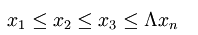则中位数就可以按下面的方式确定：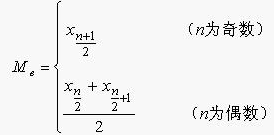例如，根据下表的数据，计算50名工人日加工零件数的中位数。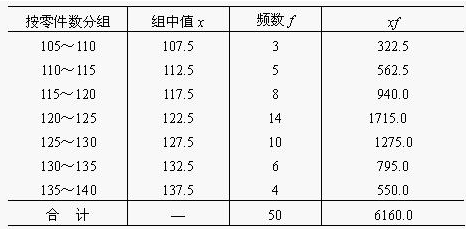中位数的位置在（50+1）/2 = 25.5，中位数在第25个数值（123）和第26个数值（123）之间，即Me = (123+123)/2=123(件)。

2、由分组资料确定中位数

由组距数列确定中位数，应先按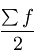的公式求出中位数所在组的位置，然后再按下限公式或上限公式确定中位数。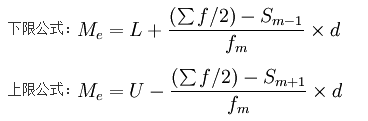公式中：

Me——中位数；

L——中位数所在组下限；

U——中位数所在组上限；

fm——为中位数所在组的次数；

——总次数；

d——中位数所在组的组距；

Sm − 1——中位数所在组以下的累计次数；

Sm + 1——中位数所在组以上的累计次数。

例：根据上面例表的数据，计算50名工人日加工零件数的中位数。

解（某企业50名工人加工零件中位数计算表）：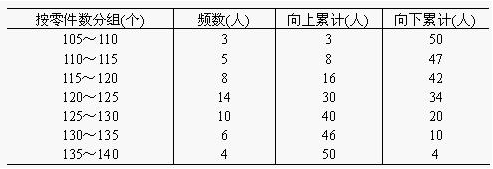由上表可知，中位数的位置=50/2=25，即中位数在120～125这一组，L=120，Sm − 1 = 16，U=125，Sm + 1 = 20，fm = 14，d=5，根据中位数公式得：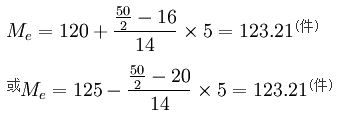3

众数是指一组数据中出现次数最多的那个数据，一组数据可以有多个众数，也可以没有众数。众数是由英国统计学家皮尔生首先提出来的。所谓众数是指社会经济现象中最普遍出现的标志值。从分布角度看，众数是具有明显集中趋势的数值。

统计上把这种在一组数据中出现次数最多的变量值叫做众数。用Mo表示。它主要用于定类（品质标志）数据的集中趋势，当然也适用于作为定序（品质标志）数据以及定距和定比（数量标志）数据集中趋势的测度值。

众数的计算：一般情况下，找出一组数据中出现次数最多的数值即可。但若所掌握的资料是组距式数列，则只能按一定的方法来推算众数的近似值。计算公式为：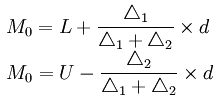公式中：

L——众数所在组下限；

U——众数所在组上限；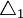——众数所在组次数与其下限的邻组次数之差；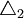——众数所在组次数与其上限的邻组次数之差；

d——众数所在组组距。

例:根据下表的数据，计算50名工人日加工零件数的众数。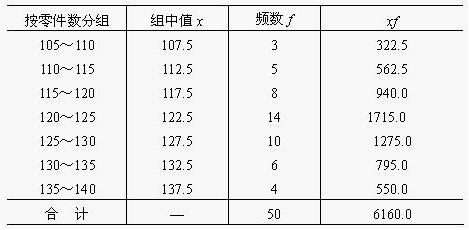解：从表中的数据可以看出，最大的频数值是14，即众数组为120~125这一组，根据公式得50名工人日加工零件的众数为：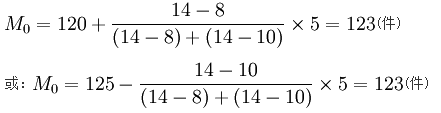众数是一种位置平均数，是总体中出现次数最多的变量值，因而在实际工作中有时有它特殊的用途。诸如，要说明一个企业中工人最普遍的技术等级，说明消费者需要的内衣、鞋袜、帽子等最普遍的号码，说明农贸市场上某种农副产品最普遍的成交价格等，都需要利用众数。但是必须注意，从分布的角度看，众数是具有明显集中趋势点的数值，一组数据分布的最高峰点所对应的数值即为众数。当然，如果数据的分布没有明显的集中趋势或最高峰点，众数也可能不存在；如果有两个最高峰点，也可以有两个众数。只有在总体单位比较多，而且又明显地集中于某个变量值时，计算众数才有意义。

如果一组数据中存在离群值，中位数和众数一般不受离群值的影响，算术平均数容易受到离群值的影响。

展开全文• rstudio中位数的公式In this tutorial, let’s learn how we can find the median in R. Median is defined as the measurement of central tendency in the data. In simpler terms, you may call it the ‘middle...

rstudio中位数的公式

In this tutorial, let’s learn how we can find the median in R. Median is defined as the measurement of central tendency in the data. In simpler terms, you may call it the ‘middle’ value.

在本教程中，让我们学习如何在R中找到中位数。中位数定义为数据中中心趋势的度量。 简单来说，您可以将其称为“中间”值。

The process includes grouping or ordering the values and then finding the middle number among them. If you encounter multiple middle values, you can take the average or ‘mean’ of those values.

该过程包括对值进行分组或排序，然后在其中找到中间的数字。 如果遇到多个中间值，则可以取这些值的平均值或“平均值”

## 中位数–优缺点 (Median – Merits and Demerits )

Merits:

优点：

• It is very easy to calculate the median. In some simple cases, you can find the median just by analyzing the values.

计算中位数非常容易。 在一些简单的情况下，您可以仅通过分析值来找到中位数。
• Median has real use in open-ended data distributions. Because the median gives more importance to the position of the number than its value.

中位数在开放式数据分发中有实际用途。 因为中位数比数字的值更重视数字的位置。
• One of the major advantages of the median is that it is not affected by the outliers present in the data.

中位数的主要优势之一是它不受数据中存在的异常值的影响。

Outliers: Outliers are described as the extreme values, which are different from the rest of the values in the data.

离群值：离群值描述为极值，与数据中的其余值不同。

Ex: The retirement age values are – (52,53,54,54,55,56,57,58,79)

例如：退休年龄值为–（52,53,54,54,55,56,57,58,79）

Here, 79 is an extreme value and it is different from the rest of the values or data. It will affect the mean and mode drastically. But Median will not be affected as it deals with position rather than the value.

在这里，79是一个极值，它不同于其余的值或数据。 它将严重影响均值和众数。 但是中位数不会受到影响，因为它只处理头寸而不是价值。

Demerits:

缺点：

• Median will not look for the accurate value as it will not utilize the entire data.

中位数不会寻找 准确的价值，因为它不会利用全部数据。
• Median is not capable of further statistical or mathematical operations.

中位数不能进行进一步的统计或数学运算。

## 查找给定值的中位数 (Finding the median of the given values)

In this section, we will create a list of values and try to find the median of those values.

在本节中，我们将创建一个值列表，并尝试查找这些值的中位数。


#creates a list
x <- c(45,76,56,87,65,45,34,56,78,98,87,65,34,48,76)

#displays the values
show(x)
---> 45 76 56 87 65 45 34 56 78 98 87 65 34 48 76

#calculates the median of the values in the list 'x'
median(x)


Output: 65

输出：65

You may wonder how 65 can be a middle value. Well, the median() function first groups or order the values in ascending or descending order, then it will calculate the middle or central value.

您可能想知道65如何成为中间值。 好吧，位数（）函数首先将值分组或以升序或降序排列，然后将计算中间值或中心值。

Note: If one or more values are found to be central values, then the average of them will be considered as the median.

注意：如果发现一个或多个值是中心值，则将它们平均值视为中位数

## 查找“国家的用电量数据”的中位数。 (Finding the median of the ‘Electricity consumption data of the countries’.)

In this section, we import the CSV file which includes the data of ‘Electricity/energy consumption’ across the above-mentioned countries – India, Romania, USA, and Jamaica in the year 2019.

在本部分中，我们导入CSV文件 ，其中包含上述国家（印度，罗马尼亚，美国和牙买加）在2019年的“电力/能源消耗”数据。

Execute the below code to find the median of the ‘Voltage’ consumed by these countries in 2019.

执行以下代码以查找这些国家/地区在2019年所消耗的“电压”中位数。

注意： 在此处查看或下载“能源消耗”数据集


#reads the value present in the file.

#displays the values.
df

#calculates the median of the 'voltage' values.
median(df$Voltage)  Output: 220 Volts, 输出：220 Note: In this data set, the results showed that the median is 220, i.e. the central tendency of the data is 220 volts. 注意：在此数据集中，结果显示中位数为220，即数据的中心趋势为220伏。 ## 借助箱形图可视化数据的中位数 (Visualizing the Median of the data with the help of the box plot) In R, you can create a box plot to understand the distribution of median as shown in the below plot. 在R中，您可以创建一个箱形图以了解中位数的分布，如下图所示。 boxplot: Boxplots are used in R to understand the distribution of data. R offers the function boxplot() to create the box graph. The thick line in the plot represents the median. boxplot R中使用Boxplots来了解数据的分布。 R提供了boxplot（）函数来创建箱形图。 图中的粗线代表中位数。 ## 使用直方图了解“电压”的中值 (Using Histogram to Understand the Median of the ‘voltage’) In this section, we are going to plot the voltage distribution with the help of a histogram in Rstudio. 在本节中，我们将借助Rstudio中的直方图来绘制电压分布 Execute the below code to plot the histogram, which shows the voltage distribution and the median of the voltage. 执行以下代码以绘制直方图，该直方图显示电压分布和电压中值。  #reads the value present in the file. df <- read.csv("energydata.csv") #displays the values. df #calculates the median of the 'voltage' values. median(df$Voltage)
#plots the histogram
hist(df$Voltage, col='orange', xlab='voltage', ylab='frequency', main='Voltage distribution') #adds the median line abline(v=median(df$Voltage), col='black', lwd='3')
legend(x='topright', c('median'),col = 'black', lwd = '3')


In the above plot, you can see the ‘black’ line, which is actually showing the median. Through the histograms we can easily demonstrate the mean, median, and density curves as well.

在上图中，您可以看到“黑”线，它实际上是显示中位数。 通过直方图，我们还可以轻松显示均值，中值和密度曲线。

## 结论 (Conclusion)

With the help of the Median() function, we can understand the central tendency of the data. Median is very easy to find in some cases, where you are able to tell the median value by just inspecting it.

借助Median（）函数，我们可以了解数据的集中趋势。 在某些情况下，很容易找到中值，您可以通过检查中值来判断中值。

R offers great visualizing functions to understand the hidden data patterns. As shown above, you can easily analyze the median using the histogram and box plots.

R提供了出色的可视化功能，以了解隐藏的数据模式。 如上所示，您可以使用直方图和箱形图轻松分析中位数

That’s all for now. Connect with us for more R tutorials. Don’t hesitate to comment below if you have any queries. Happy learning!!!.

目前为止就这样了。 与我们联系以获取更多R教程。 如有任何疑问，请在下面评论。 学习愉快！

rstudio中位数的公式

展开全文python 人工智能 大数据
• 文章目录一、中位数二、波动范围与极差三、离差、方差与标准差 一、中位数 1、中位数 将多个样本按照大小顺序排列，居于中间位置的元素为中位数 2、经典求法 1）A：样本集 2）L：样本数 3）M = (A[(L-1)/2] + A[L/2]...

### 一、中位数

1、中位数
将多个样本按照大小顺序排列，居于中间位置的元素为中位数

2、经典求法
1）A：样本集

2）L：样本数

3）M = (A[(L-1)/2] + A[L/2]) / 2

4、Numpy求法
1）对数组进行排序：np.msort(数组)

2）求中位数：M = numpy.median(数组)

5、练习

import numpy as np

# 导入数据
'0=数据源/beer_price.csv', delimiter=',',
usecols=(2, 3, 4),unpack=True
)

# 收盘价的中位数（手动求法）
sorted_prices = np.msort(closing_prices)
L = sorted_prices.size
# 运用公式M = (A[(L-1)/2] + A[L/2]) / 2
M1 = (sorted_prices[int((L-1)/2)] + sorted_prices[int(L/2)]) /2
print(M1)

# 用Numpy的median来求收盘价的中位数
M2 = np.median(closing_prices)
print(M2)


### 二、波动范围与极差

1、价格波动范围 = 最高的最高价 - 最低的最低价
range = a.max() - b.min()

2、极差 = 一个数组的最大值 - 该数组的最小值

• 经典求法：ptp = a.max() - a.min()
• Numpy求法：numpy.ptp()

3、练习

import numpy as np

# 导入数据
'0=数据源/beer_price.csv', delimiter=',',
usecols=(2, 3, 4),unpack=True
)

# 价格波动范围
range = highest_prices.max() - lowest_prices.min()
print(range)

# 极差
ptp1 = highest_prices.max() - highest_prices.min()
ptp2 = np.ptp(highest_prices)
print(ptp1, ptp2, sep='\n')


### 三、离差、方差与标准差

1、均值

• 样本：S = [s1, s2, …, sn]
• 均值：m = (s1 + s2 + … + sn) / n

2、离差： 每一个样本数据相对于平均值的偏离程度，是一个数组

• 经典求法：D = [s1-m, s2-m, …, sn-m]

3、方差： 离差平方和的平均值，是一个数

• 经典求法：v = ((s1-m)^2 + (s2-m)^2 + … + (sn-m)^2) / n
• 注意：此处/n表示总体方差和总体标准差；若是/(n-1)则是样本方差和样本标准差。当样本足够大时，二者几乎无差别，因此numpy默认计算的就是总体方差和总体标准差。

4、标准差： 方差的平方根，也叫方均根离差，是一个数

• 经典求法：std = sqrt(v)——>v是上文中求的方差
• Numpy求法：std = numpy.std(数组)
• 计算样本标准差：
std = np.std(数组, ddof=1) # ddof表示非自由样本个数

5、练习

import numpy as np

# 导入数据
'0=数据源/beer_price.csv', delimiter=',',
usecols=(4),unpack=True
)

# 计算均值、 离差、 方差、 标准差
mean_price = np.mean(closing_prices)  # 平均值
devs = closing_prices - mean_price  # 离差
var = (devs ** 2).mean()  # 方差：离差平方和的平均值
std1 = np.sqrt(var)  # 标准差
print(std1)

# 用numpy来直接算标准差
std2 = np.std(closing_prices)
print(std2)

# 计算样本标准差
std3 = np.std(closing_prices, ddof=1)
print(std3)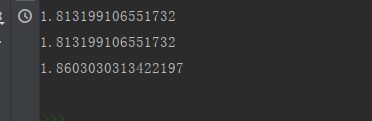展开全文python numpy 大数据
• 数据流中获取中位数需求描述需求分析C++代码如下python代码 需求描述   有一个动态的数据流，如何比较快的获得数据流的中位数。这个过程中，数据流可能会有新的数据加入。中位数定义为元素个数为奇数的序列的...算法 二叉树 python
• 否则采用中位数(四分位数间距)进行统计描述，采用非参数检验进行组间比较。大家对于四分位数间距可能会比较陌生，一般遇到数据不符合正态分布时，手足无措。今天，我们一起来看看。1四分位数(Quartile)是统计学中分...
• 调查数据分析（Survey data analysis）中经常需要计算平均数、中位数和众数。用函数编程计算40个输入数据（是取值1—10之间的任意整数）的平均数（Mean）、中位数（Median）和众数（Mode）。中位数指的是排列...c语言
• BFPRT算法是解决从n个数中选择第k大或第k小的这个经典问题的著名算法，但很多人并不了解其细节。本文将首先介绍求解这个第k小数字问题的几个思路，然后重点介绍最坏情况下复杂度仍然为O(n)的BFPRT算法。 一 ...快速排序
• 公众号后台回复“图书“，了解更多号主新书内容 作者：胖里 来源: 胖里的日常 先来看看中位数的概念。中位数（Median）又称中值，统计学中的专有名词，是按顺序排...
• ## 利用SQL求中位数(已修复BUG)

万次阅读 热门讨论 2019-09-18 16:48:36
看《SQL进阶教程》，看到用 HAVING 子句进行自连接：求中位数 这一节时对于给出的SQL不是很理解。因此花了一些时间分析了一下。体会贴此博文中。 HAVING 子句进行自连接：求中位数 中位数是指将集合中的元素按照...
• 寻找两个正序数组的中位数1、问题分析2、问题解决3、总结 1、问题分析 题目链接：https://leetcode-cn.com/problems/median-of-two-sorted-arrays/submissions/ 具体思路是： 1、 根据两个数组的总长度计算是否是 ...算法 leetcode c++
• 学习了用R计算样本数据的平均值之后（用R计算均值），下面继续学习其他统计量。 中位数 定义： 为什么要有中位数？...我们要知道的是，均值描述并不总是可靠的或最佳的。...中位数定义为数据排序位数据挖掘 大数据 R
• excel箱形图中位数 Excel中创建简单的箱形图 (Create a Simple Box Plot in Excel) A box plot (box and whisker chart) lets you show how numbers are distributed in a set of data. Excel doesn’t have a ...python excel java
• 一组数据中如果有特别大的数或特别小的数时，一般用中位数 一组数据比较多（20个以上），范围比较集中，一般用众数 其余情况一般还是平均数比较精确 一、联系与区别： 　1、平均数是通过计算得到的，因此它会因...机器学习
• 具体来说，我们将以分析历史股价为例，介绍怎样从文件载入数据，以及怎样使用NumPy的基本数学和统计分析函数。这里还将学习读写文件的方法，并尝试函数式编程和NumPy线性代数运算。 第三章 常用函数 3.9 统计分析 ...numpy
• 均值、中位数中位数的概念以及优缺点对比。偏态分布难点分析，以及偏度、峰度计算公式。文末附相关学习链接。
•  中位数：分类数据组的中间值(如果数据个数为偶数,则是两个中间数值和的一半)  众数：数据组中出现次数最多的值(或者一组值)   异常值：比几乎其他所有数字都要 大/小 很多的数值   加权平均值：对变量...四分位数 分布
• 对于未分组数据，可使用Excel的MEDIAN函数求解中位数。 对于分组数据，分为： ...假设数据在每个等级区间内均匀分布下，采用以下公式来估计组数据中位数。 看似非常简单的中位数计算，使用了实际数
• 面试时，会经常被问道，如何求解一个无序数组的中位数？很多人往往都会第一感觉就是，先将该数组排序，然后找出最中间的那个数，但是这种思路通常的时间复杂度最好是O(nlogn)，更糟的情况下会到O(n^2)，并不是最优...快速排序
• 当我深入研究时，我意识到我很难理解为给定的数据选择哪个集中趋势指标有三种:平均值，中位数和众数。 所以我决定写这篇文章来帮助像我一样这个领域里的新人来弄明白这一点，而不是害怕数据和统计。这里我们使用...
• 例如： >>> a = [8, 19, 34, 9, 18] >>> np.median(a) # 得到数组 a 的中位数 18.0 >>> np.quantile(a, 0.25) # 得到数组 a 的上四分位数 9.0 >>> np.quantile(a, 0.5) # 得到数组 a 的中位数 18.0 >>> np.quantile...python
• 于是想起数据挖掘课上提到的正偏态分布中，均值大于中位数的问题。思考很久无法证明。 关于正偏态，正态和负偏态的图如下。 正偏也叫右偏，看起来好像是峰值左，怎么会叫右偏呢？按维基百科的解释是：传统...机器学习 人工智能
• 导入数据 import pandas as pd movie= pd.read_csv('movie.csv') movie.head() Rank Title Genre Description Director ... Runtime (Minutes) Rating Votes Revenue (Millions) ...python 开发工具
•大数据 动态规划 algorithm python
• 这并不是意味着拿它相邻的单元格来替换，而是你需要寻找除了空的这个单元格，哪一行数据在其他列上的内容与存在空值的这行数据是最接近的，然后用该行的数据进行替换。这种方式较为严谨，但也比较费事。 第二种思路...
• ## C语言计算平均数/众数/中位数

千次阅读 热门讨论 2019-12-18 16:50:57
调查数据分析（Survey data analysis）中经常需要计算平均数、中位数和众数。用函数编程计算40个输入数据（是取值1—10之间的任意整数）的平均数（Mean）、中位数（Median）和众数（Mode）。中位数指的是排列...c语言
• 参考上面的草图：可以这样理解，（1）对于正偏态而言，数据大多分布右侧，从而也就把期望与中位数往右侧移动。（2）对于负偏态而言，数据大多分布左侧，从而也就把期望与中位数往左侧移动。 ...
• 对列表进行排序，然后根据长度为奇数或者偶数的不同情况计算中位数 def huahua(x): length = len(x) print(length) x.sort() print(x) if (length % 2)== 1: z=length // 2 y = x[z] else: y = (x[length//...编程语言 python
• 探索性数据分析（Exploratory Data Analysis ,EDA）是对数据进行分析并得出规律的一种数据分析方法。它是一个故事，一个数据试图讲述的故事。EDA是一种利用各种工具和图形技术(如柱状图、直方图等)分析数据的方法。 ...python
• R的统计分析通过使用许多内置函数来执行的，这些函数大部分是R基础包的一部分，并且它们将R向量与参数一起作为输入，并执行计算后给出结果。 先来看如何求平均值。 平均值是通过取数值的总和并除以数据序列...R语言...